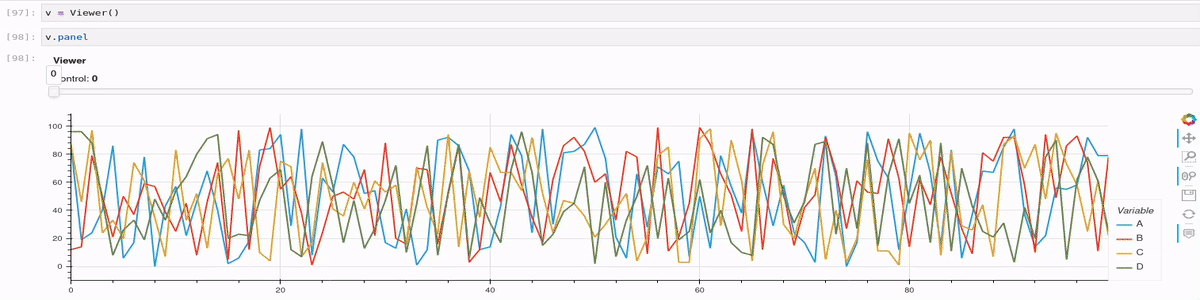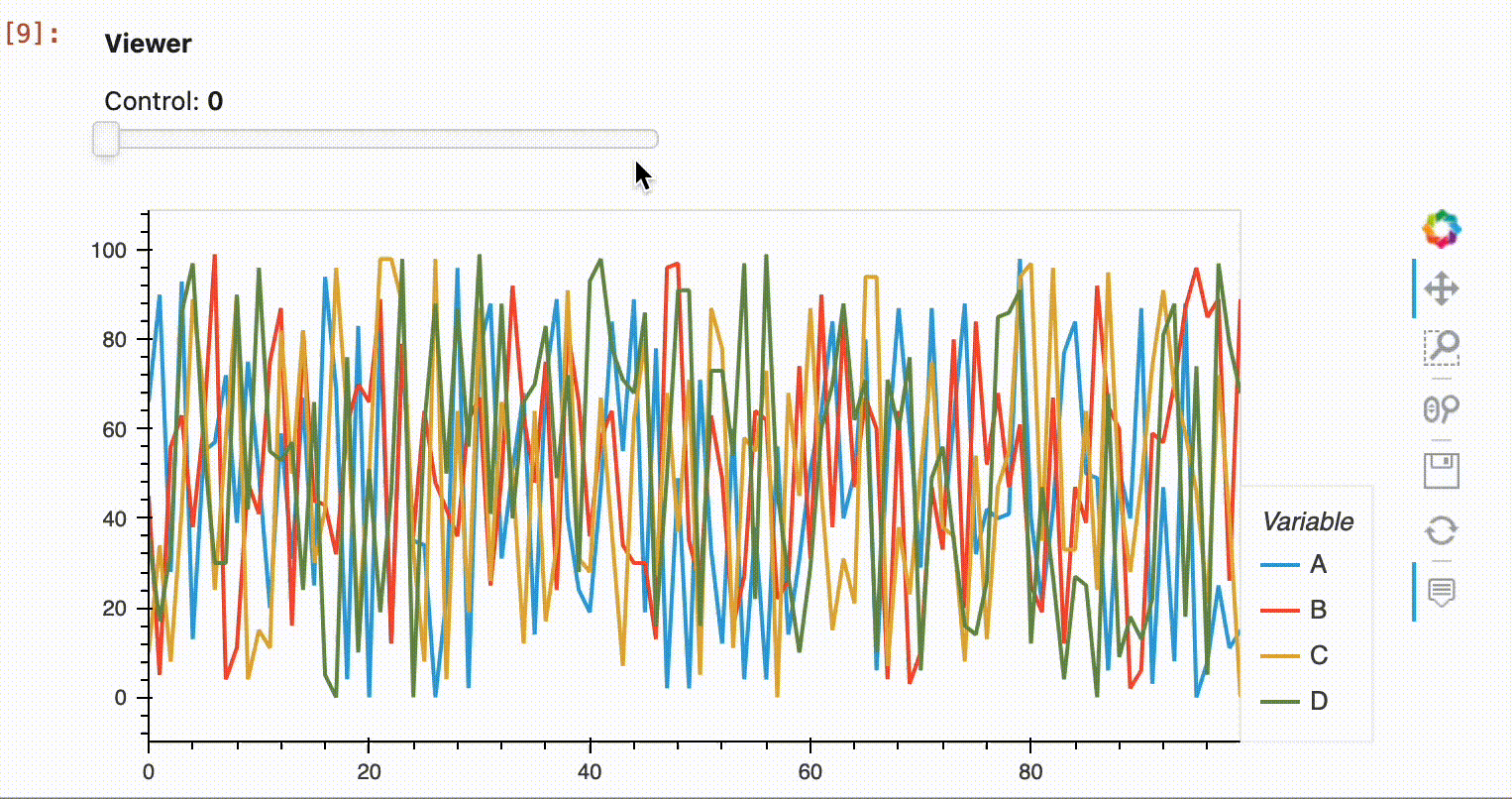# Mislabelled categories with @param.depends

Hi,

This is an example that reproduces the problem: categories can´t be updated after I change them once.
Am I supposed to do this in a fundamentally different way?

``````import panel as pn
import holoviews as hv
import hvplot.pandas  # noqa
import pandas as pd
import param
import numpy as np

class Viewer(param.Parameterized):

control = param.Number(default=0, bounds=(0,1), step=1)

def __init__(self, **params):
super().__init__(**params)

self.traces = []
for columns in ['ABCD', 'EFGH']:
self.traces.append(pd.DataFrame(np.random.randint(0,100,size=(100, 4)), columns=list(columns)))

self.panel =  pn.Column(pn.Param(self.param), pn.pane.HoloViews(hv.DynamicMap(self.view)))

@param.depends("control")
def view(self):
return self.traces[self.control].hvplot()

v = Viewer()
v.panel
``````You can notice that once I select Control=1 the categories will not revert back if I select again Control=0. The video starts with the categories A, B, C and D. It get stuck with E, F, G and H even if the actual data is correct! Hope the explanation is clear enough.

I think the below gives you what you want based on the usual methods here: Using Param with Panel — Panel v0.14.2

I must admit I was scratching my head a bit at explaining why your method gave the behaviour it did, but I think something strange goes on when you set it to the panel attribute it and call it that way. I don’t think the refreshing is done correctly on the view param method.

``````import panel as pn
import holoviews as hv
import hvplot.pandas  # noqa
import pandas as pd
import param
import numpy as np

class Viewer(param.Parameterized):

control = param.Number(default=0, bounds=(0,1), step=1)

def __init__(self, **params):
super().__init__(**params)

self.traces = []
for columns in ['ABCD', 'EFGH']:
self.traces.append(pd.DataFrame(np.random.randint(0,100,size=(100, 4)), columns=list(columns)))

@param.depends("control")
def view(self):
return self.traces[self.control].hvplot()

v = Viewer()
pn.Column(v.param, v.view)
``````My guess is that the difference is really the use of the `DynamicMap`. It might be the case (I don’t know) that `DynamicMap`s assume the dimensions are the same for all plots?